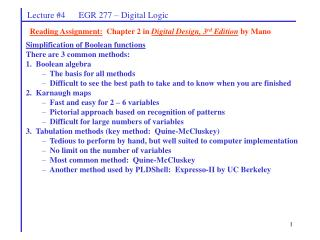DownloadDownload PresentationSimplification of Boolean functions There are 3 common methods: 1. Boolean algebra

# Simplification of Boolean functions There are 3 common methods: 1. Boolean algebra

Download Presentation## Simplification of Boolean functions There are 3 common methods: 1. Boolean algebra

- - - - - - - - - - - - - - - - - - - - - - - - - - - E N D - - - - - - - - - - - - - - - - - - - - - - - - - - -
##### Presentation Transcript

1. Lecture #4 EGR 277 – Digital Logic Reading Assignment: Chapter 2 in Digital Design, 3rd Edition by Mano Simplification of Boolean functions There are 3 common methods: 1. Boolean algebra The basis for all methods Difficult to see the best path to take and to know when you are finished 2. Karnaugh maps Fast and easy for 2 – 6 variables Pictorial approach based on recognition of patterns Difficult for large numbers of variables 3. Tabulation methods (key method: Quine-McCluskey) Tedious to perform by hand, but well suited to computer implementation No limit on the number of variables Most common method: Quine-McCluskey Another method used by PLDShell: Expresso-II by UC Berkeley

2. Lecture #4 EGR 277 – Digital Logic Karnaugh Maps (K-maps) Essentially a 2D truth table arranged in a table or map so that adjacent cells in the map differ in only one bit position. Each cell in the map corresponds to a minterm. Combining 1’s in the Kmap into groups of 1, 2, 4, 8, etc. can yield a quick minimization of a Boolean function. Kmaps are most commonly used for functions involving 2-6 variables. 2-variable K-maps The K-map has 22 = 4 cells. Example: Use a K-map to minimize the function f(x,y) = x’y + xy’ + xy

3. Lecture #4 EGR 277 – Digital Logic Example: Use a K-map to minimize each function below. 1. 2. f(x, y, z) = (0, 1, 5, 7) 3. f(x, y, z) = xyz + x’yz + x’y’z’ + y’z 4. f(a, b, c) = ab’ + ac + ab + b 3-variable K-maps The K-map has 23 = 8 cells.

4. Lecture #4 EGR 277 – Digital Logic Example: Use a K-map to minimize each function below. 4-variable K-maps The K-map has 24 = 16 cells.

5. Lecture #4 EGR 277 – Digital Logic Group size vs. number of literals: Note how the number of literals in a term is reduced by one each time the size of grouping is doubled. For the 4-variable K-map: Example: Use a K-map to minimize each function below. 1. f(A, B, C, D) = A’B’C’ + A’CD + B’CD’ + ABD + AB’D + AB’C’D’ 2. f(A, B, C, D) = B’ + C’D + BD’ + A’D + BC

6. Lecture #4 EGR 277 – Digital Logic Example: Use a K-map to minimize each function below. Identify the essential prime implicants in each problem.

7. Lecture #4 EGR 277 – Digital Logic Prime Implicants Prime Implicant – a product term which cannot be combined with other terms to yield a term with fewer literals. Hint: A prime implicant corresponds to a maximal grouping in a Kmap. Essential Prime Implicant – a prime implicant that covers at least one minterm of the function that is not covered by any other prime implicant. A good way to approach finding minimal expressions in Karnaugh maps is to find essential prime implicants first. Once those groups have been drawn on the map, it is easier to see what other groupings would most efficiently cover the rest of the minterms. In general, a function can be expressed as: F = sum of essential prime implicants + (some combination of non-essential prime implicants) Example: Identify all prime implicants and all essential prime implicants in f(A, B, C, D) = (0, 1, 3, 4, 5, 7, 11, 15)

8. Lecture #4 EGR 277 – Digital Logic 5-variable K-maps The K-map has 25 = 32 cells. Note the odd arrangement of ABCDE. This arrangement results in the left half of the K-map having the same minterm ordering as a 4-variable map. Other arrangements of ABCDE will result in different minterm ordering. Example: Use a K-map to minimize the function below. F(A, B, C, D, E) = (1, 2, 5, 6, 8, 10, 12, 14, 17, 18, 21, 22, 24, 26, 28, 30)

9. Lecture #4 EGR 277 – Digital Logic 6-variable K-maps The K-map has 26 = 64 cells. Note the odd arrangement of ABCDEF. This arrangement results in the top half of the K-map having the same minterm ordering as a 5-variable map. Other arrangements of ABCDEF will result in different minterm ordering.

10. Lecture #4 EGR 277 – Digital Logic Example: Use a K-map to minimize the function below. F(A, B, C, D, E, F) = (2, 3, 7, 10, 11, 16, 17, 20, 21, 34, 35, 38, 39, 42, 43, 46, 47, 50-55, 58, 59, 62, 63)